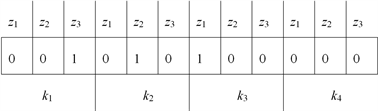﻿ 智慧城市中无线网络成本最优节点部署机制

# 智慧城市中无线网络成本最优节点部署机制Optimal Node Deployment Scheme for Wireless Network in Smart City

Abstract: As one of the key problems addressed in the application of wireless sensor networks (WSNs), sensor deployment is the significant methods of guaranteeing the quality of service in networks. In this paper, from the optimal node deployment, we introduce and analyze two kinds of algorithm to solve the optimal solutions.

1. 引言

2. 遗传算法

1) 选择初始生命种群；

2) 循环：评价种群中的个体适应度；以比例原则(分数高的挑中的概率也较高)选择下一种群；通过交叉和变异改变种群；

3) 直到停止循环的条件满足。Figure 1. Chromosome design

$\begin{array}{l}{p}_{1}=\left\{{g}_{11},{g}_{12},\cdots ,{g}_{1|N||K|}\right\},\\ {p}_{2}=\left\{{g}_{21},{g}_{22},\cdots ,{g}_{2|N||K|}\right\},\\ \text{\hspace{0.17em}}\text{\hspace{0.17em}}\text{\hspace{0.17em}}\text{\hspace{0.17em}}\text{\hspace{0.17em}}\text{\hspace{0.17em}}\text{\hspace{0.17em}}\text{\hspace{0.17em}}\text{\hspace{0.17em}}\text{\hspace{0.17em}}\text{\hspace{0.17em}}\text{\hspace{0.17em}}\text{\hspace{0.17em}}\text{\hspace{0.17em}}\text{\hspace{0.17em}}⋮\\ {p}_{i}=\left\{{g}_{i1},{g}_{i2},\cdots ,{g}_{i|N||K|}\right\},\\ \text{\hspace{0.17em}}\text{\hspace{0.17em}}\text{\hspace{0.17em}}\text{\hspace{0.17em}}\text{\hspace{0.17em}}\text{\hspace{0.17em}}\text{\hspace{0.17em}}\text{\hspace{0.17em}}\text{\hspace{0.17em}}\text{\hspace{0.17em}}\text{\hspace{0.17em}}\text{\hspace{0.17em}}\text{\hspace{0.17em}}\text{\hspace{0.17em}}\text{\hspace{0.17em}}⋮\\ {p}_{s}=\left\{{g}_{s1},{g}_{s2},\cdots ,{g}_{s|N||K|}\right\},\end{array}$

$f=\underset{i=1}{\overset{N}{\sum }}\underset{k=1}{\overset{K}{\sum }}{c}_{ik}{x}_{ik}+M\left\{\mathrm{min}{\left\{0,\left\{\underset{i=1}{\overset{N}{\sum }}\underset{k=1}{\overset{K}{\sum }}{a}_{ijk}{x}_{ik}-{b}_{i}\right\}\right\}}^{2}\right\}$

3. 粒子群优化算法

${V}_{ij}\left(t+1\right)=W{V}_{ij}\left(t\right)+{c}_{1}r\left({p}_{ij}\left(t\right)-{x}_{ij}\left(t\right)\right)+{c}_{2}r\left({g}_{ij}\left(t\right)-{x}_{ij}\left(t\right)\right)$ (1)

${x}_{ij}\left(t+1\right)={x}_{ij}\left(t\right)+{V}_{ij}\left(t+1\right)$

${V}_{ij}=\left\{\begin{array}{l}{V}_{\mathrm{max}},\text{}{V}_{i}>{V}_{\mathrm{max}},\text{}\\ -{V}_{\mathrm{max}}\text{,}{V}_{i}<-{V}_{\mathrm{max}}.\end{array}$

${x}_{ij}\left(t+1\right)=\left\{\begin{array}{l}1,r (2)

${x}_{ij}$ 取1或0的概率， ${V}_{ij}$ 越大，则 ${x}_{ij}$ 取1的概率越大。 ${V}_{\mathrm{max}}$ 则能够限制 ${x}_{ij}$ 取1或0的概率， ${V}_{\mathrm{max}}$ 值越大，则算法接近于当前最优解附近的局部搜索，相反，则算法越接近于全局搜索。为了避免 $S\left({V}_{ij}\right)$ 太接近于0.0或1.0，可以通过限制 ${V}_{ij}$ 的大小来实现。具体过程为：起始阶段，对粒子进行编码以及粒子群初始化，初始化粒子群的参数 $w,{c}_{1},{c}_{2}$ 。在 $\omega$ 中引入自适应扰动机制，增强粒子群跳出局部最优的能力

$\omega ={\omega }_{\mathrm{max}}-\frac{{\omega }_{\mathrm{max}}-{\omega }_{\mathrm{min}}}{ite{r}_{\mathrm{max}}}×iter×{\left[1-\frac{g}{2{g}_{\mathrm{max}}}\right]}^{g}$ (3)

$g=\left\{\begin{array}{l}0,若最优解在进化\\ g+1,若最优解已G次没有进化,且g+1\le {g}_{\mathrm{max}},\\ {g}_{\mathrm{max}},其他情况.\end{array}$ (4)

$f=\underset{i=1}{\overset{N}{\sum }}\underset{k=1}{\overset{K}{\sum }}{c}_{ik}{x}_{ik}+M\left\{\mathrm{min}{\left\{0,\left\{\underset{i=1}{\overset{N}{\sum }}\underset{k=1}{\overset{K}{\sum }}{a}_{ijk}{x}_{ik}-{b}_{i}\right\}\right\}}^{2}\right\}$ (5)

4. 结论

 Li, F. and Wang, Y. (2008) Gateway Placement for Throughput Optimization in Wireless Mesh Networks. Mobile Networks and Applications, 13, 198-211.
https://doi.org/10.1007/s11036-008-0034-8

 Seyedzadegan, M., Othmanet, M., Mohd, B.A., et al. (2013) Zero-Degree Algorithm for Internet Gateway Placement in Backbone Wireless Mesh Networks. Journal of Network and Computer Applications, 36, 1705-1723.
https://doi.org/10.1016/j.jnca.2013.02.031

 Durocher, S., Jampani, K.R., Lubiw, A., et al. (2011) Modeling Gateway Placement in Wireless Networks: Geometric K-Centers of Unit Disc Graphs. Computational Geometry, 44, 286-302.
https://doi.org/10.1016/j.comgeo.2010.12.003

 Lee, G. and Murray, A.T. (2010) Maximal Covering with Network Survivability Requirements in Wireless Mesh Networks. Computers, Environment and Urban Systems, 34, 49-57.
https://doi.org/10.1016/j.compenvurbsys.2009.05.004

 Aoun, B., Boutaba, R. and Iraqi, Y. (2006) Gateway Placement Optimization in WMN with QoS Constraints. IEEE Journal on Selected Areas in Communications, 24, 2127-2136.
https://doi.org/10.1109/JSAC.2006.881606

Top